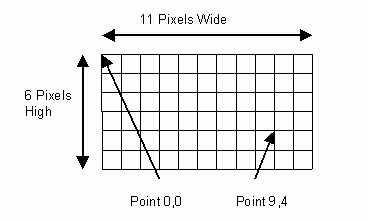PointsWhen you draw you need a space to draw on to. We call this space a Canvas. A Canvas is made up of tiny colored blocks called pixels. Typically a pixel is about 1/72th of an inch square. You can specify how many pixels wide and high your Canvas is. If you are drawing onto the Canvas you need to specify where you want your drawing to take place. You do this by giving the distance from the top left of the Canvas. You need a horizontal offset and a vertical offset (often called the x and y coordinates respectively). These offsets are measured in numbers of pixels starting at zero. Together these two coordinates are called a Point. The point at the top left of a Canvas is always 0,0 and is often called the Origin.Often you need to use groups of points to specify what you want drawn and where. Say you want to draw a line. To describe where you it to be you need to state a start Point and an end Point. Many shapes are described using sets of Points. For instance, a rectangle only needs two Points to describe it (just like the line).